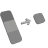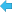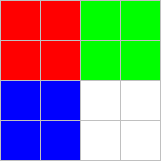Back to Adaptive Vision Studio website

You are here: Start » Filter Reference » Image Pixel Statistics » ImageSumImageSum

Computes the sum of the image pixel values.

Name Type DescriptioninImage Image Input imageinRoi Region* Range of pixels to be processedoutSumColor PixeloutSumValue Real

Examples

Assume, that the subject of the analysis is 4x4 image made of pixels in different colors - red, green, blue and white:Red (255, 0, 0) Green (0, 255, 0) Blue (0, 0, 255) White (255, 255, 255)

On the output outSumColor the sum of pixel values for each channel of an image will be computed.
For above example it will be R, G and B components, but it is also possible to use another color spaces.
Sum of color for R channel will be: $$4 * 255 + 4 * 255 = 2040$$ - sum of four red pixels and four red-components from white pixels.
Similarly, sum of colors for G channel and B channel will also be: $$4 * 255 + 4 * 255 = 2040$$
Finally, the result for above example will be 1x4 dimensional vector [2040, 2040, 2040, 0]. Notice, that zero is present in result vector, because RGB color space has only three components.

On the output outSumValue the average value of pixel of the image will be calculated according to the following formula:
Sum of elements of outSumColor / number of components in color space

Therefore, he result for above example will be $$\frac{2040 + 2040 + 2040} {3} = \frac{6120}{3} = 2040$$

Remarks

When only a part of an image should be processed use inRoi input to specify region of interest.

Hardware Acceleration

This operation supports automatic parallelization for multicore and multiprocessor systems.

Complexity Level

This filter is available on Advanced Complexity Level.